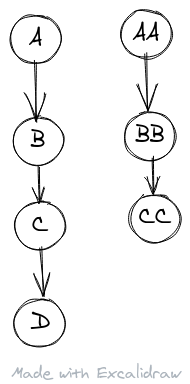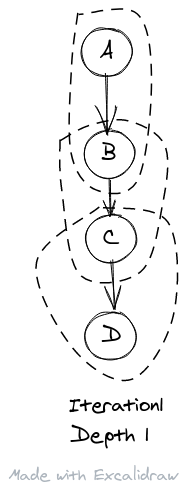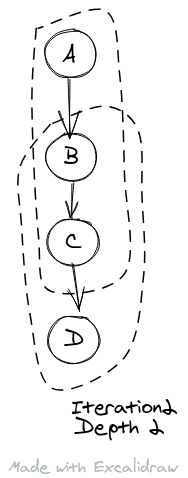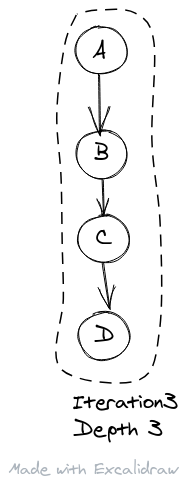# An Unnecessarily Long-Winded Introduction to the Essence of Datalog

+++

In this post, I’ll gradually build up to a naive implementation of the Datalog engine in Python. An updated version of this post can be found here

+++

## Datalog Concepts

We can have simple `facts` in our database. e.g. Bob is a man. Abe is a man. In Datalog, we write it as:

``````man("Bob")
man("Abe")``````

`man` is like a table in a database. `man("Bob")` is a relation in that table. We’ll also call it a `base` relation.

Next, we create the business logic i.e. `rules`.

Someone is a person if he is a man `person(X) :- man(X)`

`person(X)` is a `derived` relation, as it is derived from some base relation `man(X)`. This is similar to a view in the database.

`X` is a `logical variable`. So `man(X)` could be used to refer to all `man` relations in the database.

``````from typing import *
from dataclasses import dataclass
from pprint import pprint``````

We can create a logical variable like this:

``````@dataclass(frozen=True)
class Variable:
name: str``````
``X = Variable('X')``

A relation could be:

`man("Bob")`

`parent("John", "Chester")` # John is a parent of Chester

It could also be components of a rule e.g. `man(X)` or `person(X)` in `person(X) :- man(X)`

So a relation has a name(e.g. `parent`) and a list of attributes(e.g. `"John"` and `"Chester"` or `X`).

``````@dataclass(frozen=True, eq=True)
class Relation:
"""
man("Bob) is Relation("man", ("Bob",)) # ("Bob",) is a single valued tuple
parent("John", "Chester") is Relation("parent", ("John", "Chester"))
man(X) is Relation("man", (Variable("X"),))

"""
name: str
attributes: Tuple``````

A rule could be:

• `person(X) :- man(X)` i.e. `X` is a person if he is man.
• `father(X, Y) :- man(X), parent(X, Y)` i.e. `X` is a father of `Y` if `X` is a man and `X` is the parent of `Y`

A rule has:

• a head relation which is on the left of the `:-` symbol e.g. `person(X)` and `father(X, Y)` above
• a body of relations which is on the right of the `:-` symbol e.g. `man(X)` and `man(X), parent(X, Y)` above

Since Datalog is declarative, the order of the relations in the body does not matter. Both the statements below have the same meaning:

`father(X, Y) :- man(X), parent(X, Y)`

`father(X, Y) :- parent(X, Y), man(X)` # reversing the order does not matter

So, the body can be represented as a set

``````@dataclass(frozen=True, eq=True)
class Rule:
body: Set[Relation]``````

The last element of Datalog is the query. The simplest query is no rules, just facts.

Given:

``````man("Abe)
man("Bob")
woman("Abby")``````

A query could be: `man(X)` # Find me all men

The query should return: `{man("Bob"), man("George")}`

This would be similar to a SQL query `select * from man`

+++

## Simple Relation Query

+++

The simplest query is to find a matching relation. Taking the above example, let’s code that up in Python.

``````X = Variable('X')
abe = Relation("man", ("Abe",))
bob = Relation("man", ("Bob",))
abby = Relation("woman", ("Abby",))
database = {abe, bob, abby}
no_rules = []
query = Relation("man", (X,))``````

For some function `run_simplest`, I expect: `assert run_simplest(database, no_rules, query) == {abe, bob}`

The simplest run would iterate through all the facts and filter those facts that match the query by relation name.

``````def name_match(fact: Relation, query: Relation) -> bool:
return fact.name == query.name

def filter_facts(database: Set[Relation], query: Relation, match: Callable) -> Set[Relation]:
return {fact for fact in database if match(fact, query)}

def run_simplest(database: Set[Relation], rules: List[Rule], query: Relation) -> Set[Relation]:
return filter_facts(database, query, name_match)

assert run_simplest(database, no_rules, query) == {abe, bob}``````

Let’s add some facts of length two:

``````parent("Abe", "Bob") # Abe is a parent of Bob
parent("Abby", "Bob")
parent("Bob", "Carl")
parent("Bob", "Connor")
parent("Beatrice", "Carl")``````

I may want to query who are the parents of Carl

`parent(X, "Carl")` should return `{parent("Bob", "Carl"), parent("Beatrice", "Carl")}`

`parent(X, "Carl")` is similar to `select * from parent where child = "Carl"` if there was a table `parent` with columns `parent` and `child`)

The beauty of Datalog is that you can ask the inverse without additional code e.g. Who are the children of Bob

`parent("Bob", X)` should return `{parent("Bob", "Carl"), parent("Bob", "Connor")}`

Let’s code that up. Also from now on, I’m going to make a helper functions to make it easy to express relations like the lambda `parent` below.

``````parent = lambda parent, child: Relation("parent", (parent, child))
database = {
parent("Abe", "Bob"), # Abe is a parent of Bob
parent("Abby", "Bob"),
parent("Bob", "Carl"),
parent("Bob", "Connor"),
parent("Beatrice", "Carl")
}``````

Now, to the implementation. For a query to match, an argument at position N in the query should match the argument at position N in the fact. For e.g `assert query_variable_match(parent("A", "Bob"), parent(X, "Bob") ) == True`

Logical variables are special. They get a free pass like `X` above.

``````def query_variable_match(fact: Relation, query: Relation) -> bool:
if fact.name != query.name:
return False

# TODO: zip is duplicated?
for query_attribute, fact_attribute in zip(query.attributes, fact.attributes):
if not isinstance(query_attribute, Variable) and query_attribute != fact_attribute:
return False
return True

assert query_variable_match(parent("A", "Bob"), parent(X, "Bob") ) == True
assert query_variable_match(parent("A", "Bob"), parent("A", X)) == True
assert query_variable_match(parent("A", "NoMatch"), parent(X, "Bob") ) == False

def run_with_filter(database: Set[Relation], rules: List[Rule], query: Relation) -> Set[Relation]:
return filter_facts(database, query, query_variable_match)``````

So does it work?

``````parents_carl =  run_with_filter(database, [], parent(X, "Carl"))
assert parents_carl == {parent("Bob", "Carl"), parent("Beatrice", "Carl")}

children_bob =  run_with_filter(database, [], parent("Bob", X))
assert children_bob == {parent("Bob", "Carl"), parent("Bob", "Connor")}``````

## Simple Rule Query

Let’s add a rule to our program.

``human(X) :- man(X) # You are human if you are man.``

An example database below:

``````man("Bob")
man("George")
animal("Tiger")``````

Query:

`human(X)` # Find me all humans

The query should return: `{human("Bob"), human("George")}`

``````man = lambda x: Relation("man", (x,))
animal = lambda x: Relation("animal", (x,))
human = lambda x: Relation("human", (x,))
X = Variable("X")

body = [man(X)]
human_rule = Rule(head, body) # No pun was intended
database = {
man("Abe"),
man("Bob"),
animal("Tiger")
}
rules = [human_rule]
query = human(X)``````

For each rule, for each relation in it’s body, if it matches with any of the facts in the database, then get the attributes of that fact and create a derived relation with those attributes. E.g., since we have `man("Abe")` and our rule `human(X) :- man(X)`, we add a derived relation to our database `human("Abe")`

``````def match_relation_and_fact(relation: Relation, fact: Relation) -> Optional[Dict]:
if relation.name == fact.name:
return dict(zip(relation.attributes, fact.attributes))

def match_relation_and_database(database: Set[Relation], relation: Relation) -> List[Dict]:
inferred_attributes = []
for fact in database:
attributes = match_relation_and_fact(relation, fact)
if attributes:
inferred_attributes.append(attributes)
return inferred_attributes

def evaluate_rule_simple(rule: Rule, database: Set[Relation]) -> Set[Relation]:
relation = list(rule.body) # For now, our body has only one relation
all_matches = match_relation_and_database(database, relation)
# We use the Python feature below that if we call `values` on a dictionary,
# it will preserve the order that was given when the dictionary was created
# i.e. in the `zip` inside `match_relation_and_database`. Thank God.
return {Relation(rule.head.name, tuple(attributes.values())) for attributes in all_matches}``````

This `evaluate_rule_simple` can be passed to a function which will `evaluate` it on each rule for the database to generate the final knowledge base.

``````def generate_knowledgebase(evaluate: Callable, database: Set[Relation], rules: List[Rule]):
knowledge_base = database
for rule in rules:
evaluation = evaluate(rule, database)
knowledge_base = knowledge_base.union(evaluation)
return knowledge_base ``````

And finally, we have

``````def run_rule_simple(database: Set[Relation], rules: List[Rule], query: Relation):
knowledge_base = generate_knowledgebase(evaluate_rule_simple, database, rules)
return filter_facts(knowledge_base, query, query_variable_match)

# Test Cases
simplest_rule_result = run_rule_simple(database, rules, query)
assert simplest_rule_result == {human("Abe"), human("Bob")}, f"result was {simplest_rule_result}"``````

## Logical AND Query

+++

Next, we introduce logical AND(conjunction). i.e. Given

``````parent("Abe", "Bob"), # Abe is a parent of Bob
parent("Abby", "Bob"),
parent("Bob", "Carl"),
parent("Bob", "Connor"),
parent("Beatrice", "Carl"),
man("Abe"),
man("Bob"),
woman("Abby"),
woman("Beatrice")``````

We’d like to find all the fathers in the house. A person is a father if he is a parent and he is a man. i.e. `father(X, Y) :- parent(X, Y), man(X)`

``````X = Variable("X")
Y = Variable("Y")
woman = lambda x: Relation("woman", (x,))
father = lambda x, y: Relation("father", (x, y))

database = {
parent("Abe", "Bob"), # Abe is a parent of Bob
parent("Abby", "Bob"),
parent("Bob", "Carl"),
parent("Bob", "Connor"),
parent("Beatrice", "Carl"),
man("Abe"),
man("Bob"),
woman("Abby"),
woman("Beatrice")
}

father_rule = Rule(father(X, Y), {parent(X, Y), man(X)})
rules = [father_rule]
query = father(X, Y)``````

How does the match change? We need to add logic for the conjunction i.e. when we match the body to the facts, we have to check if the attributes of the facts match across the entire body, e.g. for the body `parent(X, Y), man(X)`

• parent(“Abe”, “Bob”), man(“Abe”) is a match as there is a common value `Abe` across the entire body at same place as `X`.
• parent(“Abby”, “Bob”) does not match as there is no `man("Abby")`.

Let’s first code up this common value logic as `has_common_value`. We also have to start pairing variables to values e.g. `{'X': 'Abe'}`

This combination below:

`has_common_value({ X: 'Abe', Y: 'Bob'}, {X: 'Abe'})` should return `True`

``````def has_common_value(attrs1: Dict[Variable, Any], attrs2: Dict[Variable, Any]) -> bool:
common_vars = set(attrs1.keys()).intersection(set(attrs2.keys()))
return all([attrs1[c] == attrs2[c] for c in common_vars])``````

Once we have that, we know that `match_relation_and_database` will return as before, a list of body attributes which each match a fact in the database. It’s time to conjunct. We may get some input like:

``````[[{X: 'Bob', Y: 'Carl'},    # <= All facts that match parent(X,Y)
{X: 'Beatrice', Y: 'Carl'},
{X: 'Abe', Y: 'Bob'},
{X: 'Abby', Y: 'Bob'},
{X: 'Bob', Y: 'Connor'}],
[{X: 'Bob'},               # <= All facts that match man(X)
{X: 'Abe'}]]``````

For the body `man(X), parent(X, Y)`, we expect back from a function `conjunct`:

``````[{X: 'Bob', Y: 'Carl'},
{X: 'Abe', Y: 'Bob'},
{X: 'Bob', Y: 'Connor'}]``````

Just hacking it for now.

``````def conjunct(body_attributes: List[List[Dict]]) -> List:
# TODO: Does not cover body lengths greater than 2
result = []
if len(body_attributes) == 1:
return body_attributes

attr1 = body_attributes
attr2 = body_attributes
for a1 in attr1:
for a2 in attr2:
_c = has_common_value(a1, a2)
if _c:
result.append({**a1, **a2})
return result``````

I also realized that though the body can return many attributes which have ‘conjuncted’, we only need those which are in the head. e.g. for a rule `relation1(X) :- relation2(X,Y), relation3(X)`, `relation1` just needs `X` so I’ll just pull that.

``````def rule_attributes(relation: Relation, attr: Dict[Variable, Any]) -> Tuple:
return tuple([attr[a] for a in relation.attributes])``````

So the final `evaluate` function becomes

``````def evaluate_logical_operators_in_rule(rule: Rule, database: List[Relation]) -> Set[Relation]:
body_attributes = []

for relation in rule.body:
_attributes = match_relation_and_database(database, relation)
body_attributes.append(_attributes)

attributes = conjunct(body_attributes)

def run_logical_operators(database: Set[Relation], rules: List[Rule], query: Relation):
knowledge_base = generate_knowledgebase(evaluate_logical_operators_in_rule, database, rules)
return filter_facts(knowledge_base, query, query_variable_match)``````

Let’s test that it works for single relation bodies, preventing any regressions.

``````simple_conjunct_rules = [Rule(human(X), {man(X)})]
assert run_logical_operators(database, simple_conjunct_rules, human(X)) == {human("Abe"), human("Bob")}``````

And our final test

``assert run_logical_operators(database, rules, query) == {father("Abe", "Bob"), father("Bob", "Carl"), father("Bob", "Connor")}``

## Logical OR Query

+++

Logical OR is just specifying two separate rules with the same head. E.g.

``````human(X) :- man(X)
human(X) :- woman(X)``````

In Python, given:

``````database = {
animal("Tiger"),
man("Abe"),
man("Bob"),
woman("Abby"),
woman("Beatrice")
}

man_rule = Rule(human(X), {man(X)})
woman_rule = Rule(human(X), {woman(X)})
rules = [man_rule, woman_rule]
query = human(X)

assert run_logical_operators(database, rules, query) == {
human("Abe"),
human("Bob"),
human("Abby"),
human("Beatrice")
}``````

## Recursive Relations Query

+++

Next, we introduce the reason why we are interested in Datalog. Datalog intuitively captures hierarchies or recursion. E.g. we want to find all who are ancestors of someone. Given:

``````parent("A", "B") # A is the parent of B
parent("B", "C")
parent("C", "D")
parent("AA", "BB")
parent("BB", "CC")``````

A parent X of Y is by definition an ancestor.

`ancestor(X, Y) :- parent(X, Y)`

If you are a parent of Y and Y is an an ancestor, then you are an ancestor as well.

`ancestor(X, Z) :- parent(X, Y), ancestor(Y, Z)`

Query: `ancestor(X, Y)` should return all the parents above as ancestors

``````ancestor("A", "B") # A is the ancestor of B
ancestor("A", "C") # A -> B -> C
ancestor("A", "D") # A -> B -> C -> D
ancestor("B", "C")
ancestor("B", "D") # B -> C -> D
ancestor("C", "D")
ancestor("AA", "BB")
ancestor("AA", "CC") # AA -> BB -> CC
ancestor("BB", "CC")``````

In Python,

``````ancestor = lambda ancestor, descendant: Relation('ancestor', (ancestor, descendant))

database = {
parent("A", "B"),
parent("B", "C"),
parent("C", "D"),
parent("AA", "BB"),
parent("BB", "CC")
}

X = Variable("X")
Y = Variable("Y")
Z = Variable("Z")
# ancestor(X, Y) :- parent(X, Y)
ancestor_rule_base = Rule(ancestor(X, Y), [parent(X, Y)])
# ancestor(X, Z) :- parent(X, Y), ancestor(Y, Z)
ancestor_rule_recursive = Rule(ancestor(X, Z), {parent(X, Y), ancestor(Y, Z)})

rules = [ancestor_rule_base, ancestor_rule_recursive]``````

Alright, let’s dive into this. What is different from `run_logical_operator`? It’s the hierarchy or recursion. If you see it as hierarchy(I’m visualizing this as a tree), one has to keep on going until we reach the top of the tree.So let’s imagine how we would process the above example. In the first pass, we would do the simplest inference from base fact to derived fact using the base rule of `ancestor(X, Y) :- parent(X, Y)`.

Showing one hierarchy as an example(starting from `A`).``````Pass 1: Base Facts and Inferred facts i.e. KnowledgeBase1
parent("A", "B"),
parent("B", "C"),
parent("C", "D"),
parent("AA", "BB"),
parent("BB", "CC")]
# ----------------- New inferred facts below --------------
ancestor("A", "B"),
ancestor("B", "C"),
ancestor("C", "D"),
ancestor("AA", "BB"),
ancestor("BB", "CC")``````

Now that’s done, we can focus on inference from a combination of inferred facts and base facts to new inferred facts using the recursive rule `ancestor(X, Z) :- parent(X, Y), ancestor(Y, Z)`. For e.g. in `KnowledgeBase1`, we have `parent("C","D")` and `ancestor("B", "C")` , so we can infer the fact `ancestor("B", "D")` i.e grandparents. We keep on doing this till we get:``````Pass 2: KnowledgeBase2
parent("A", "B"),
parent("B", "C"),
parent("C", "D"),
parent("AA", "BB"),
parent("BB", "CC")
ancestor("A", "B"),
ancestor("B", "C"),
ancestor("C", "D"),
ancestor("AA", "BB"),
ancestor("BB", "CC")
# ----------------- New inferred facts below --------------
ancestor("A", "C")
ancestor("B", "D")
ancestor("AA", "CC")``````

Do we stop? No, we have to keep on going till we find all the ancestors. Let’s apply the rules to `KnowledgeBase2` and get``````Pass 3: KnowledgeBase3
parent("A", "B"),
parent("B", "C"),
parent("C", "D"),
parent("AA", "BB"),
parent("BB", "CC")
ancestor("A", "B"),
ancestor("B", "C"),
ancestor("C", "D"),
ancestor("AA", "BB"),
ancestor("BB", "CC")
ancestor("A", "C")
ancestor("B", "D")
ancestor("AA", "CC")
# ----------------- New inferred facts below --------------
ancestor("A", "D")``````

i.e `A` is the great grand parent of `D`

Do we stop? Yes(if you look at the above example), but the computer does not know that. There could be new inferred facts, so let’s try again for `KnowledgeBase4`.

``````Pass 4: KnowledgeBase4
parent("A", "B"),
parent("B", "C"),
parent("C", "D"),
parent("AA", "BB"),
parent("BB", "CC"),
ancestor("A", "B"),
ancestor("B", "C"),
ancestor("C", "D"),
ancestor("AA", "BB"),
ancestor("BB", "CC")
ancestor("A", "C")
ancestor("B", "D")
ancestor("AA", "CC")
ancestor("A", "D")
# ----------------- New inferred facts below --------------
No New Facts``````

Aha! There are no more new inferred facts. If we do another pass on `KnowledgeBase4`, it would come out the same. So we can stop!

So the logic to stop would be: Take the output of each iteration. If it matches the input to that iteration, stop(as we did not learn any new inferred facts). If not a match, then run another iteration. Let’s call this method `iterate_until_no_change`.

``````def iterate_until_no_change(transform: Callable, initial_value: Set) -> Set:
a_input = initial_value

while True:
a_output = transform(a_input)
if a_output == a_input:
return a_output
a_input = a_output``````

Now, we already have `evaluate_logical_operators_in_rule`. That will be our `transform` function above. So putting this all together below.

``````def run_recursive(database: Set[Relation], rules: List[Rule], query: Relation):
transformer = lambda a_knowledgebase: generate_knowledgebase(evaluate_logical_operators_in_rule, a_knowledgebase, rules)
knowledgebase = iterate_until_no_change(transformer, database)
return filter_facts(knowledgebase, query, query_variable_match)``````

Let’s define the query

``````query = ancestor(X, Y)

recursive_result = run_recursive(database, rules, query)

expected_result = {
ancestor("A", "B"),
ancestor("B", "C"),
ancestor("C", "D"),
ancestor("AA", "BB"),
ancestor("BB", "CC"),
ancestor("A", "C"),
ancestor("B", "D"),
ancestor("AA", "CC"),
ancestor("A", "D")
}

assert recursive_result == expected_result, f"{recursive_result} not equal to {expected_result}"``````

Let’s explore other queries we can ask. Is `AA` the ancestor of `C`?(No! Such an impolite question)

``````query = ancestor("AA", "C")

assert run_recursive(database, rules, query) == set()``````

What if I want to find all ancestors of `C`?

``````query = ancestor(X, "C")
assert run_recursive(database, rules, query) == {ancestor("A", "C"), ancestor("B", "C")}``````

What if I want to find who all are the descendants of `AA`. Again, use the same query but just reverse the order!

``````query = ancestor("AA", X)
assert run_recursive(database, rules, query) == {ancestor("AA", "BB"), ancestor("AA", "CC")}``````

Finally, who are the intermediates between `A` and `D` i.e. `B` and `C`.

`Z` is an intermediate of `X` and `Y` if `X` is it’s ancestor and `Y` is its descendant.

``intermediate(Z, X, Y) :- ancestor(X, Z), ancestor(Z, Y)``

In Python,

``````intermediate = lambda intermediate, start, end: Relation("intermediate", (intermediate, start, end))
intermediate_body = {ancestor(X, Z), ancestor(Z, Y)}

rules = [ancestor_rule_base, ancestor_rule_recursive, intermediate_rule]
query = intermediate(Z, "A", "D")

assert run_recursive(database, rules, query) == {intermediate("B", "A", "D"), intermediate("C", "A", "D")}``````

+++

That’s it! If you found it interesting, all these ideas will be explored in Mercylog, a soon to be built Datalog inspired library in Python.

• One aspect of Datalog being declarative is that the order of rules does not matter either. So technically, instead of `rules = [rule1, rule2]`, we could have used `rules = frozenset([rule1, rule2])`. The latter is a bit more clutter so I used simple lists.